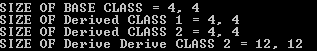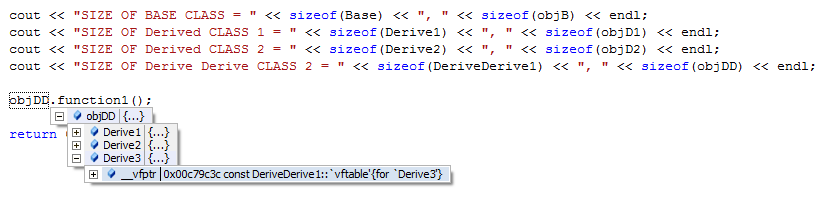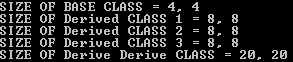# C & C++ Programming - Size Of & VPTR

```    class Base
{
public:
virtual void function1()
{}
};

class Derive1: public Base
{
public:
virtual void function1()
{}
};

class Derive2: public Base
{
public:
virtual void function1()
{}
};

class Derive3
{
public:
virtual void function1()
{}
};

class DeriveDerive1: public Derive1, Derive2, Derive3
{
public:
virtual void function1()
{
cout << __FUNCTION__ << endl;
}
};

int main()
{
Base objB;
Derive1 objD1;
Derive2 objD2;
DeriveDerive1 objDD;

cout << "SIZE OF BASE CLASS = " << sizeof(Base) << ", " << sizeof(objB) << endl;
cout << "SIZE OF Derived CLASS 1 = " << sizeof(Derive1) << ", " << sizeof(objD1) << endl;
cout << "SIZE OF Derived CLASS 2 = " << sizeof(Derive2) << ", " << sizeof(objD2) << endl;
cout << "SIZE OF Derive Derive CLASS = " << sizeof(DeriveDerive1) << ", " << sizeof(objDD) << endl;
}
```The class DeriveDerive is derived from 3 classes, each having virtual functions thus having individual VTABLE for each.
It could be observed the sizeof objDD is 12. For the fact 3 VTABLE pointers are associated with object objDD to access each base class VTABLE.```    class DeriveDerive1: virtual public Derive1, Derive2, Derive3
{
public:
virtual void function1()
{
cout << __FUNCTION__ << endl;
}
};
```
Modifying the code as above will result in sizeof objDD = 16. 4 more is added to accomodate BPTR (Base Table pointer).
```    class Base
{
public:
virtual void function1()
{}
};

class Base2
{
public:
virtual void function1()
{}
};

class Derive1: virtual public Base
{
public:
virtual void function1()
{}
}; // Size of Derive1 = 4 + 4; VPTR, BPTR

class Derive2: virtual public Base
{
public:
virtual void function1()
{}
};

class Derive3 : virtual public Base2
{
public:
virtual void function1()
{}
};

class DeriveDerive1:  public Derive1, Derive2, Derive3
{
public:
virtual void function1()
{
cout << __FUNCTION__ << endl;
}
};  // Size of Derive1 = 4 + 4 + 4, 4 + 4; 2VPTR, BPTR; VPTR + BPTR;
// This is derived from 3 different class, of which 2 virually devired from same class and one from diffrent.

////////////////////////////////////////////////////////////////////////////////////////////////////////////////
// WATCH

-		objDD	{...}	DeriveDerive1
-		Derive1	{...}	Derive1
-		    Base	{...}	Base
-		        __vfptr	0x0033991c const DeriveDerive1::`vftable'{for `Derive1'}	*
	0x003310af DeriveDerive1::function1(void)	*

-		Derive2	{...}	Derive2
-		    Base	{...}	Base
-		        __vfptr	0x0033991c const DeriveDerive1::`vftable'{for `Derive1'}	*
	0x003310af DeriveDerive1::function1(void)	*

-		Derive3	{...}	Derive3
-		    Base2	{...}	Base2
-		        __vfptr	0x003398f4 const DeriveDerive1::`vftable'{for `Derive3'}	*